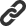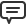### Blog Options

#### Archive

<< February 2020 >>

# Blog

All Blog Posts  |  Next Post  |  Previous Post

#New symbolic math features in TMS Analytics & Physics library

Bookmarks:

#### Wednesday, October 17, 2018

TMS Analytics & Physics developing library provides many useful and unique features for working with symbolic math expressions. New version 2.6 introduces new capabilities and improvements for symbolic manipulations with math formula.

First great innovation is that the partial derivative operator can be used in math formulae as an implicit operation. This means there is no need to evaluate symbolic expression for some derivative and then use the expression in some math formula. The derivative can be used in formula implicitly. For example, it is possible to use the following expression in formulae ‘?sin(x^2)/?x’. The derivative by ‘x’ evaluated by the system implicitly and used when required for other operations.
Some examples of syntactically correct expressions with the derivative operator:

∂²(x^2*sin(a*x))/∂x²
∂²(y^2+1-e^-x)/∂x∂y
∂sinh(x*y)/∂x-∂ln(y/x)/∂y

Implicit derivative operator supports mixed and high-order derivatives (up to 9-th order). Using the operator allows write math expressions in short and readable form without substituting explicit expression for the derivative, which could be more complicated in common case.
Here is an example code for calculating value of an expression, containing implicit derivative operator:

```var
f: string;
v: TValue;
begin
f:= 'e^(b*x)*(∂y^2/∂y)-∂sin(a*x)/∂x';
v:= translator.Calculate(f);
// code for using ‘v’ value...
end;
```

The code for the expression calculation is simple and does not require explicit derivative expression – all operations done by the evaluation machine in the background. All other methods, such as simplification and derivative, are applicable for expression with the operator. Let us consider the following example:

```var
f1, f2, df1, df2: string;
begin
f1:= 'a*x^2+∂²(sin(a*x)*e^(b*y))/∂x∂y';
df1:= translator.Derivative(f1, 'x');

f2:= 'a*x^2+∂²(sin(a*x)*e^(b*y))/∂a∂b';
df2:= translator.Derivative(f2, 'x');

// code for using symbolic derivatives ‘df1’ and ‘df2’...
end;
```

Result symbolic expressions for ‘df1’ and ‘df2’ are the following:

df1 = a*2*x+∂³(sin(a*x)*e^(b*y))/∂x;²∂y
df2 = a*2*x+∂²(cos(a*x)*a*e^(b*y))/∂a∂b

This shows that symbolic operations applied to the implicit derivative operator with some features and the expressions still contain the operator (see documentation for full information about the features). The new method ‘Explicit’ of the Translator class can be used to get explicit symbolic expression for any formula, containing implicit operations. Here is the example code:

```var
f, ef: string;
begin
f:= 'e^(b*x)*(∂y^2/∂y)-∂sin(a*x)/∂x';
ef:= translator.Explicit(f);
// code for using explicit symbolic expression ‘ef’...
end;
```
The explicit symbolic expressions for the example is the following: ef = e^(b*x)*(2*y)-cos(a*x)*a Another great feature of the version is functional operators. They are like ‘user defined’ functions and allow introducing new functionality without writing new classes or adding new packages. New function can be introduced with the ‘AddFunction’ method. Here is the code of a simple example:
```var
f: string;
v: TValue;
begin

f:= 'S(a)-S(a/2)';
v:= translator.Calculate(f);
// code for using ‘v’ value...
end;```
First, we added new function (functional operator) with name ‘S’, one argument ‘r’ and the expression for circle area formula ‘Pi*r^2’. As the function introduced it can be called in symbolic expressions as other functions with different arguments. In the example code the function used to calculate area of the ring with outer radius ‘a’ and inner radius ‘a/2’. Functional operators not restricted with simple formula and can include many arguments and any acceptable expressions as function formula or arguments. For example:

```var
f: string;
v: TValue;
begin

f:= 'Series(e^y y)';
v:= translator.Calculate(f);
// code for using ‘v’ value...
end;```

The introduced function ‘Series’ is the Tailor’s series with degrees up to fourth for F(x). The function accepts two arguments: function ‘F’ and variable ‘x’. The function then used to calculate ‘approximate’ value of ‘e^y’ represented with the series.
Using functional operators together with implicit derivative operator allows defining differential operators, such as gradient, Laplacian and other. Let us consider the following code:

```var
f, ef: string;
begin

f:= 'Laplace(A*sin(x)*y^2+B*cos(x)*e^-y x y)';
ef:= translator.Explicit(f);
ef:= translator.Simplify(ef);
// code for using explicit symbolic expression ‘ef’...
end;```

The introduced function ‘Laplace’ is the 2D Laplacian differential operator for function ‘F’ and variables ‘x’ and ‘y’. The function used for evaluating symbolic expression of Laplacian for the math expression ‘A*sin(x)*y^2+B*cos(x)*e^-y’. After simplification the result expression is ‘-A*sin(x)*y^2+A*sin(x)*2’. It should be noted here that the result does not contain the ‘B’ variable. It is because Laplacian for the part ‘B*cos(x)*e^-y’ gives zero.
Thus, all new symbolic capabilities allow using TMS Analytics & Physics library to build applications like Computer Algebra Systems (CAS). The version 2.6 is already released now. Source code of the example application can be downloaded from here.

Bruno Fierens

Bookmarks: